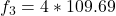A guitar string 0.65 m long has a tension of 61 N and a mass per unit length of 3.0 g/m. (i) What is the speed of waves on the string when i

Question

A guitar string 0.65 m long has a tension of 61 N and a mass per unit length of 3.0 g/m. (i) What is the speed of waves on the string when it is plucked? (ii) What is the string’s fundamental frequency of vibration when plucked? (iii) At what other frequencies will this string vibrate?

in progress 0
5 months 2021-08-18T14:37:04+00:00 1 Answers 19 views 0

i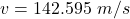ii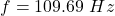iii1 )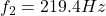iii2)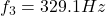iii3)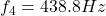Explanation:

From the question we are told that

The length of the string is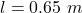The tension on the string is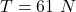The mass per unit length is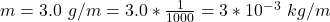The speed of wave on the string is mathematically represented as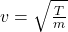substituting values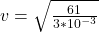generally the  string’s  frequency is mathematically represented as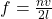n = 1  given that the frequency we are to find is the fundamental frequency

So

substituting values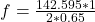The  frequencies at which the string would vibrate include

1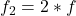Here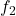is  know as the second harmonic and the value is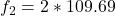2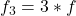Here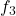is  know as the third harmonic and the value is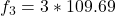3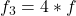Here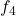is  know as the fourth harmonic and the value is